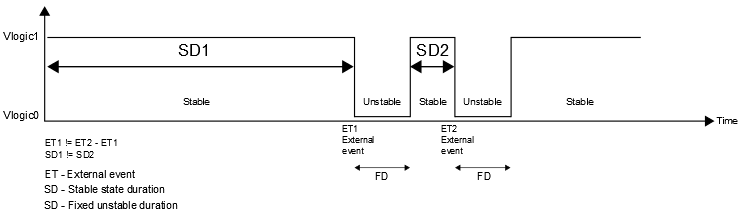### Multivibrator as a state machine

#### It's pretty hard to imagine, but a multivibrator is one of the most common terms used in the electronic world. How many times did you use a PWM signal to control the rotational speed of a DC motor? Did you ever think about a CPU clock generator? Well, a PWM signal as well as the CPU clock generator belongs to the class of a multivibrator. In this blog post, you will see that a multivibrator is a square wave generator modeled by a state machine.

Multivibrator classes

There are three types of multivibrators. Classification is related to a state stability. But, before I continue with the classification, I would like to say a few words about nature of a multivibrator. A multivibrator is a state machine with two discrete states and a two transitions in between. Generally speaking, with a two states and a two transitions we are able to construct a three different types of a multivibrators independent of an implementation:
1. monostable multivibrator
2. bistable multivibrator and
3. astable multivibrator.
The general goal of a multivibrator machine is to be a stable. For a multivibrator with an unstable state, stability could be achieved through a direct transition to another state. A direct transition type does not depend on any condition as an external transition type (triggered by some of the external events). An external transition type could be triggered by a human hand or by the light source for example.

A general (generic) state machine

In order for the state machine to be constructed, only a bit of information is required for encoding two different states. Let's say that the first state is encoded with a logic 1 and a second state is encoded with a logic 0 (below picture). Each of the logic states has electrical voltage counterpart marked as Vlogic1 and Vlogic0 respectively in the following text.Monostable, bistable and astable multivibrator respectively.

As you can see, we have three state machines with the same states and different transitions in between. Monostable multivibrator has one external transition and one direct transition. Bistable multivibrator has two external transitions while an astable multivibrator has two direct transitions. Everything else is the same for all three state machines. By transition from one state to another state, we are able to generate the sequence of the logic numbers (101010101010...) and the counterpart square wave signal.

There are two types of square wave periods: the stable and unstable. The transition event occurrence, determines the duration of the each stable period, presented in the below picture. For simplicity, the unstable period has a fixed duration.

Monostable square wave generator

As it is already defined, the external event occurrence determines duration of the stable period. That means, that successive stable periods could be different. Please look at a difference between ET2 and ET1. The period ET2 is less than the period ET1, |ET2 - ET1| != |ET1|. In that case, conclusion is simple, stable periods have a different durations, |SD1| != |SD2|.Monostable square wave signal

At the first occurrence of an external event ET1, the monostable square generator change its state from the stable to the unstable state which result in the signal changes from Vlogic1 to Vlogic0 voltage value. After the fixed period, the monostable square generator goes directly to the stable state raising signal voltage from Vlogic0 to Vlogic1 value, and stay there until the next external event ET2.

The next external event is triggered at time ET2, which results in different duration of the stable periods: SD1 and SD2. As you can see, successive stable periods could be different regarding time of the external event occurrence.

Bistable square wave generator

Bistable square wave generator does not have a direct transition like the monostable generator. It has two external events which mean that each duration of the stable period could be different, as it is presented in the below picture.Bistable square wave signal

Astable square wave generator

Astable state machine is the unstable machine which always search state of the stability. Because astable state machine does not have a stable state, the stability is not possible to achieve. The unstable nature generates the oscillation signal with frequency F. Frequency F is determined by the duration of the state T, F = 1 / T. Astable state machine is the most general way to produce microprocessor clock or the pulse width modulated signal used for the speed DC motor control.Astable square wave signal

YouTube video: example of the bistable multivibrator.